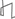Research Abstracts Home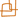CSAIL Digital Archive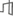Research Activities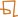CSAIL HomeResearch Abstracts - 2007IntroductionPerception & LearningPhysical, Biological & Social SystemsSystemsTheory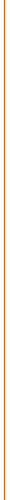### Huge Networks, Tiny Faulty Nodes

#### Enoch Peserico & Larry Rudolph

##### Scalable Computing and Scalable Networking

Computing models such as Cellular Automata are truly scalable: cells of = a fixed complexity can form regular lattices capable of carrying out arbitr= ary computations. We explore similar scalability issues in the context of n= etworking, where the goal is not to obtain an output from an input, but to = have any pair of nodes of a network communicate efficiently. Does there exi= st a "universal" networking node, with a fixed amount of resources (in ter= ms of memory, reliability etc.) that can be used to form efficient networks= of arbitrary size and topology? Can one achieve robust connectivity and ef= ficient bandwidth utilization in the presence of finite reliability (i.e. a= s networks grow without individual components becoming more reliable)? Can = one route along almost optimal paths with routing tables that do not grow w= ith the size of the network?

##### Scalable Robust Connectivity

Can one achieve good connectivity on networks where links and/or nodes f= ail with a constant probability p, even at distances much larger than the a= verage distance between failures, 1/p? With good connectivity we mean that = regions of the network are connected by almost as many disjoint, entirely n= on-faulty paths as if the network was entirely free of faults. We show that= this is indeed possible, regardless of the distance between the two region= s, as long as they are connected by a strip of width at least logarithmic i= n their distance, and without too many large "holes" (a formally defined co= ncept, roughly corresponding to the intuition of 2 dimensional holes). Hole= s, in the presence of faults, can seriously compromise connectivity in the = region "around" them, effectively eroding it up to a distance proportional = to the logarithm of the "circumference" of the hole times the probability o= f a fault. Our results  put in question some networking conventional = wisdom and practices - for example, mobile/ad hoc networking simulations wo= uld give more robust results if performed on Sierpinski gaskets rather than= simple squares or circles, and the simple number of disjoint paths connect= ing two regions can be a poor predictor of the resilience of their connecti= vity in the presence of faults.

##### Ultra Compact Routing

Can a network route messages along paths of length within a constant fa= ctor of the optimal, using routing tables that hold only a constant number = of bits/node? The answer has long been known to be negative : there exis= t some graphs on which routing tables of size polynomial in the number of n= odes in the network are necessary to achieve a constant path stretch. We sh= ow  how to achieve a constant path stretch using only a constant number = of bits per node (less than a node needs to even store its own unique ident= ifier!) by accepting only a very mild, "natural" restriction on the topolog= y of the network: that the number of nodes within a region should grow at m= ost polynomially with the diameter of the network. This condition is met by= any network physically implementable with nodes of size bounded away from = zero and links of finite length.

##### References:

 David Peleg and Eli Upfal, A Tradeoff between Space and Efficiency for Routing Tables. In Proceedings of STOC 1988, pp. 43--52.

 Enoch Peserico. Huge Networks, Tiny Faulty Nodes. PhD Thesis,=20 MIT CSAIL 2007.

 Enoch Peserico and Larry Rudolph.=20 Robust Network Connectivity: when it's the big picture that matters. In Proceedings of SIGMETRICS/Performance 2006, pp. 299--310.Computer Science and Artificial Intelligence Laboratory (CSAIL) The Stata Center, Building 32 - 32 Vassar Street - Cambridge, MA 02139 - USA tel:+1-617-253-0073 - publications@csail.mit.edu